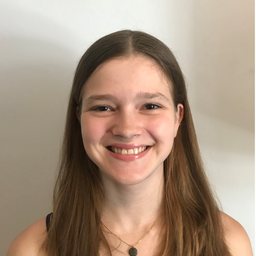# In mathematics, a compound inequality is an inequality involving two or more inequalities connected by logical operators such as "and" and "or". Compound inequalities can be written in many ways. For example: 2x+3 or 5x+5 >10

#### Topics

No Related Subtopics

### Discussion

You must be signed in to discuss.
##### Top Educators##### Catherine R.

Missouri State University### Recommended Quiz

#### Algebra

Create your own quiz or take a quiz that has been automatically generated based on what you have been learning. Expose yourself to new questions and test your abilities with different levels of difficulty.

### Video Transcript

So in this example, we're being asked to write the compound in the quality for the given freeze. Then we're going to draft the solution set on the number line. So the given free is that we have. It says that all real numbers that are greater than or equal to seven or less than negative five. So it might be helpful to start by graphing this on the number line in order to help us create our inequality. So the first thing is, well, what values air really important here? What are key values? Well, that would be seven and negative five. And remember, when you put them on the number line, the smaller of the two has to go on the left. So we have to put negative five first and then seven on the right, and you could put values in between if you'd like. That's perfectly fine. All right, let's start with the first part of this freeze. It says We're talking about all real numbers that are greater than or equal to seven. Well, does that mean sevens included? Well, it is because it says it could be greater than or equal to seven. So in terms of our number line, we're gonna have a close circle at seven. Well, where? The values that are greater than or equal to seven. Well, they would be to the right, So we're going to shade the right hand side of our number line. So any values in this red shaded area will make the first part of our sentence true, because all of these values are greater than or equal to seven. So now let's take a look at the second part of our sentence. It says that these real numbers could be also less than negative five. Well, does that mean negative? FIEs included. It's not strictly has to be less than negative five, Which means that it negative five on a number line will have an open circle. Now, where are values that are less than negative? Five? Well, they would be to the left. So we're on our number line. We're gonna shade to the left of negative five because any of the numbers in the shaded area are all values that are all real numbers. I should say that are less than negative five. So now we've graft our solution on the number line. Now we need to write inequality statement to represent this number line, which in fact, represents our original phrase. So let's start by writing a number in the inequality to represent the first half of our number line. Well, these values of X so again always start with a variable, and then our key numbers negative. Five. Well, remember these air less than negative five. So we'll have a less than sign on. We put the or equal to part because negative five is not included in the solution set. Now let's write inequality to represent the right half. So again we'll start with X. We know we're gonna end with our key value of seven, and these values are all greater than seven or they could be equal to it, so we'll use a greater than or equal to sign. And the Onley. Everything that you need to include is the word. Or so you always want to make sure you include that word in your, um, compound inequality. So now we have written our compound in the quality to represent the phrase X is less than negative. Five or X is greater than or equal to seven now, just so you know, the order that you put your two inequalities doesn't matter. So you could have also written this kind of going on in the order that your original phrase set you could have also written this as X is greater than equal to seven or X is less than negative five. Either way is perfectly fine.Syracuse University
##### Top Algebra Educators##### Catherine R.

Missouri State University